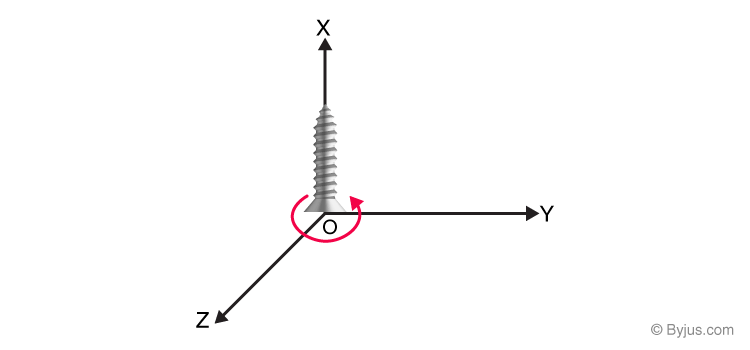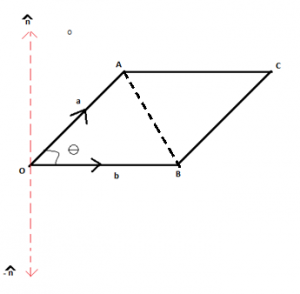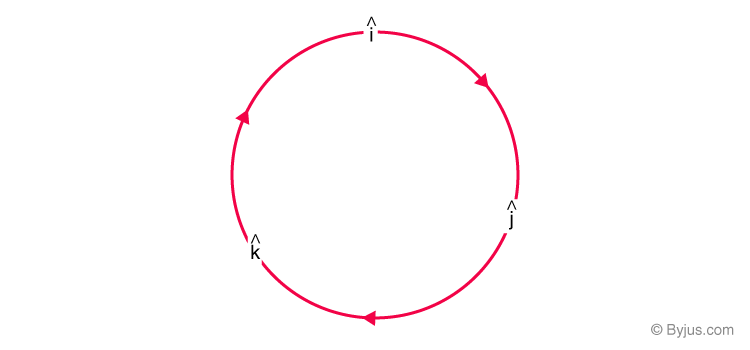# Vector Product

A vector is an object that has both the direction and the magnitude. The length indicates the magnitude of the vectors, whereas the arrow indicates the direction. There are different types of vectors. In general, there are two ways of multiplying vectors.

(i) Dot product of vectors (also known as Scalar product)

(ii) Cross product of vectors (also known as Vector product).

In the upcoming discussion, we will focus on Vector product i.e. the cross product of vectors.

## Cross product of Vectors (Vector Product)

The vector product of two vectors a and b is given by a vector whose magnitude is given by

$$\begin{array}{l}|a||b|sin\theta\end{array}$$
$$\begin{array}{l}(where \; 0^\circ \leq \theta \leq 180^\circ)\end{array}$$
which represents the angle between the two vectors and the direction of the resultant vector is given by a unit vector
$$\begin{array}{l}\hat{n}\end{array}$$
whose direction is perpendicular to both the vectors a and b in such a way that
$$\begin{array}{l}a, b~ and~ \hat{n}\end{array}$$
are oriented in right-handed system.

Right-handed orientation means that if vector a is turned in the direction of vector b then the direction of the unit vector

$$\begin{array}{l}\hat{n} \end{array}$$
will be in the direction in which a right-handed screw would rotate if moved in a similar manner. Also, both the given vectors a and b are not null vectors and non-parallel in nature. The right-hand thumb rule gives an idea of the direction of the resultant vector.Thus we can say that,

$$\begin{array}{l}a × b\end{array}$$
=
$$\begin{array}{l}|a||b|sin\theta ~\hat{n}\end{array}$$
, where
$$\begin{array}{l}a × b \end{array}$$
represents the cross product of two vectors. If in any case, any of the vectors is null or both the vectors are parallel to each other, then the cross product is not defined.

In such a situation we can say that

$$\begin{array}{l}a × b\end{array}$$
=
$$\begin{array}{l}0\end{array}$$

## Physical Representation of Vectorsa and b are the adjacent sides of the parallelogram OACB  and

$$\begin{array}{l}\theta\end{array}$$
is the angle between the vectors a and b. Then the area of the parallelogram is given by
$$\begin{array}{l}|a × b|\end{array}$$
=
$$\begin{array}{l}|a||b|sin\theta\end{array}$$
.

## Properties of Vector Product

i) The vector product is do not have Commutative Property. It is given by,

$$\begin{array}{l}a × b\end{array}$$
= –
$$\begin{array}{l}(b × a)\end{array}$$

ii) The following property holds true in case of vector multiplication:

$$\begin{array}{l}(ka)× b \end{array}$$
=
$$\begin{array}{l}k(a × b)\end{array}$$
=
$$\begin{array}{l} a × (kb)\end{array}$$

iii) If the given vectors are collinear then

$$\begin{array}{l}a × b \end{array}$$
=
$$\begin{array}{l}0\end{array}$$

(Since the angle between both the vectors would be 0, then sin 0 = 0)

iv) Following the above property

We can say that the vector multiplication of a vector with itself would be

$$\begin{array}{l}a × a \end{array}$$
=
$$\begin{array}{l}|a||a|sin 0 ~\hat{n} \end{array}$$
=
$$\begin{array}{l} 0\end{array}$$

Also in terms of unit vector notation

$$\begin{array}{l}\hat{i} × \hat{i} = \hat{j} × \hat{j} = \hat{k} × \hat{k}\end{array}$$
=
$$\begin{array}{l} 0\end{array}$$

From the above discussion it also follows that

$$\begin{array}{l}\hat{i} × \hat{j} = \hat{k} = -\hat{j} × \hat{i}\end{array}$$

$$\begin{array}{l} \hat{j} × \hat{k} = \hat{i} = -\hat{k} × \hat{j}\end{array}$$

$$\begin{array}{l}\hat{k} × \hat{i} = \hat{j} = -\hat{i} × \hat{k}\end{array}$$

This can be easily represented using the following diagram. On moving in clockwise direction and taking cross product of any two pair of the unit vectors we get the third one and in anticlockwise direction we get the negative resultant.v) a × b in terms of unit vectors can be represented as

$$\begin{array}{l} a\end{array}$$
=
$$\begin{array}{l} a_1 \hat{i} + a_2 \hat{j} + a_3 \hat{k} \end{array}$$

$$\begin{array}{l} b\end{array}$$
=
$$\begin{array}{l} b_1 \hat{i} + b_2 \hat{j} + b_3 \hat{k} \end{array}$$

Then

$$\begin{array}{l}\overrightarrow{a} × \overrightarrow{b}\end{array}$$
=
$$\begin{array}{l} (a_1 \hat{i} + a_2 \hat{j} + a_3 \hat{k})(b_1 \hat{i} + b_2 \hat{j} + b_3 \hat{k})\end{array}$$

Expanding we will get

$$\begin{array}{l}|a||b|sin\theta ~\hat{n}\end{array}$$
=
$$\begin{array}{l}(a_2b_3 – a_3b_2)\hat{i} + (a_3b_1 – a_1b_3)\hat{j} + (a_1b_2 – a_2b_1)\hat{k}\end{array}$$

This can also be given by making use of determinants as shown,

$$\begin{array}{l}a × b \end{array}$$
=
$$\begin{array}{l}\begin{vmatrix} \hat{i} & \hat{j} & \hat{k}\cr a_1 & a_2 & a_3 \cr b_1 & b_2 & b_3 \end{vmatrix}\end{array}$$

vi) Distributive Law:

$$\begin{array}{l}a × (b + c)\end{array}$$
=
$$\begin{array}{l}a × b + a × c\end{array}$$
<

Hence now we know how to calculate the vector product of given vectors. To know more about vectors and other concepts in Mathematics please visit our website www.byjus.com and fall in love with learning.